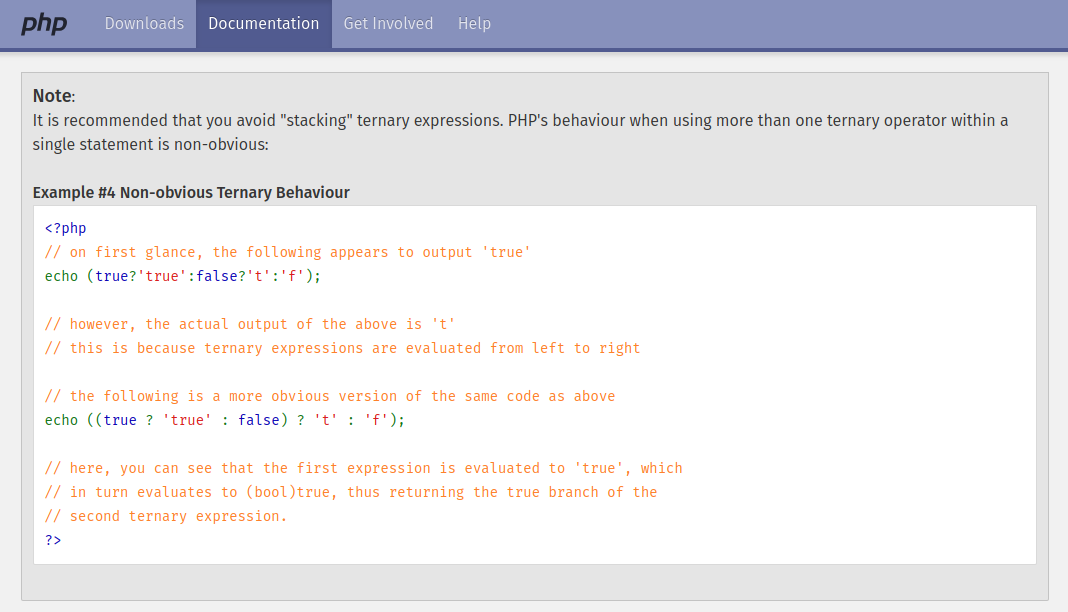Last Updated: October 27, 2018
·
216
· jasny# Stacking the Ternary Operator in PHP

###### php operator inline

TLDR;

``````\$grade =
(\$score >= 90 ? 'A' : null) ??
(\$score >= 75 ? 'B' : null) ??
(\$score >= 65 ? 'C' : null) ??
(\$score >= 55 ? 'D' : null) ??
'F';``````

In PHP the ternary operator is left-associative and therefore behaves entirely incorrectly:As a solution, you could use parentheses, but this quickly becomes messy

``\$grade = (\$score >= 90 ? 'A' : (\$score >= 75 ? 'B' : (\$score >= 65 ? 'C' : (\$score >= 55 ? 'D' : 'F') ) ) );``

I prefer to have a single condition per line and not have to worry about the parenthesis.

As a workaround, we can use the Null Coalescing (`??`) operator, given that the value that is selected will never be `null`.

``````\$grade =
(\$score >= 90 ? 'A' : null) ??
(\$score >= 75 ? 'B' : null) ??
(\$score >= 65 ? 'C' : null) ??
(\$score >= 55 ? 'D' : null) ??
'F';``````

In this example, we're just using static strings. However, this also works as expected if we were calling functions for the value, as only the function of the matching condition is called.

CAVEAT; if the value is `null` the next condition is tried, rather than returning `null`.